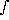i,calc = B-1(Ri,calc)
Ri,calc =i Bi(Ts) +'i Hi'i(ps) cos(0) +Bi(T(p)) (dui/dln p) (dln p) +iui(ps)Bi(T(p)) (ddi/dln p) (dln p)

Bi(T) is the Planck function evaluated at the effective channel wavenumber.
Hi is the channel averaged solar irradiance at the top of the atmosphere.0 is the local zenith angle of the Sun.'i is the channel averaged two path transmittance from the Sun to the surface to the satellite.ui(p) is the atmospheric transmittance measured between p and the top of the atmosphere for channel i.di(p) is the atmospheric transmittance between p and the surface for channel i.

Explicit retrieved parametersi is the surface emissivity ati.
Ts is the surface temperature.
T(p) is the surface temperature profile.i is the surface spectral reflectivity ati.'i is the surface spectral bidirectional reflectance of solar radiation ati.

Implicit retrieved parameters ( i.e., withini and'i).
CO2(p) is the carbon dioxide profile.
q(p) is the humidity (water) profile.
O3(p) is the ozone profile.
CO(p) is the carbon monoxide profile.
CH4(p) is the methane profile.
NO2(p) is the nitrogen dioxide profile.